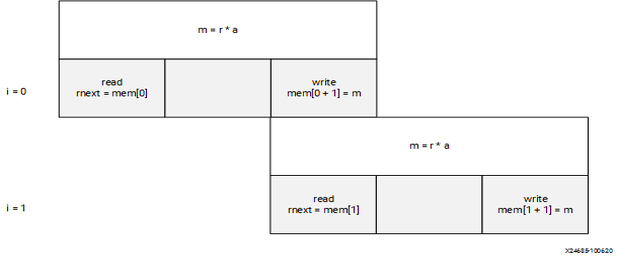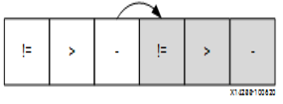# Managing Pipeline Dependencies - 2022.2 English

## Vitis High-Level Synthesis User Guide (UG1399)

Document ID
UG1399
Release Date
2022-12-07
Version
2022.2 English

Vitis HLS constructs a hardware datapath that corresponds to the C/C++ source code. When there is no pipeline directive, the execution is always sequential and so there are no dependencies that the tool needs to take into account. But when features of the design has been pipelined, the tool needs to ensure that any possible dependencies are respected in the hardware that Vitis HLS generates.

Typical cases of data dependencies or memory dependencies are when a read or a write occurs after a previous read or write.

• A read-after-write (RAW), also called a true dependency, is when an instruction (and data it reads/uses) depends on the result of a previous operation.
• I1: t = a * b;
• I2: c = t + 1;

The read in statement I2 depends on the write of t in statement I1. If the instructions are reordered, it uses the previous value of t.

• A write-after-read (WAR), also called an anti-dependence, is when an instruction cannot update a register or memory (by a write) before a previous instruction has read the data.
• I1: b = t + a;
• I2: t = 3;

The write in statement I2 cannot execute before statement I1, otherwise the result of b is invalid.

• A write-after-write (WAW) is a dependence when a register or memory must be written in specific order otherwise other instructions might be corrupted.
• I1: t = a * b;
• I2: c = t + 1;
• I3: t = 1;

The write in statement I3 must happen after the write in statement I1. Otherwise, the statement I2 result is incorrect.

• A read-after-read has no dependency as instructions can be freely reordered if the variable is not declared as volatile. If it is, then the order of instructions has to be maintained.

For example, when a pipeline is generated, the tool needs to take care that a register or memory location read at a later stage has not been modified by a previous write. This is a true dependency or read-after-write (RAW) dependency. A specific example is:

``````int top(int a, int b) {
int t,c;
I1: t = a * b;
I2: c = t + 1;
return c;
}``````

Statement `I2` cannot be evaluated before statement `I1` completes because there is a dependency on variable `t`. In hardware, if the multiplication takes 3 clock cycles, then `I2` is delayed for that amount of time. If the above function is pipelined, then Vitis HLS detects this as a true dependency and schedules the operations accordingly. It uses data forwarding optimization to remove the RAW dependency, so that the function can operate at II =1.

Memory dependencies arise when the example applies to an array and not just variables.

``````int top(int a) {
int r=1,rnext,m,i,out;
static int mem;
L1: for(i=0;i<=254;i++) {
#pragma HLS PIPELINE II=1
I1:     m = r * a; mem[i+1] = m;    // line 7
I2:     rnext = mem[i]; r = rnext; // line 8
}
return r;
}``````

In the above example, scheduling of loop `L1` leads to a scheduling warning message:

``````WARNING: [SCHED 204-68] Unable to enforce a carried dependency constraint
(II = 1, distance = 1) between 'store' operation (top.cpp:7) of variable 'm', top.cpp:7
on array 'mem' and 'load' operation ('rnext', top.cpp:8) on array 'mem'.
INFO: [SCHED 204-61] Pipelining result: Target II: 1, Final II: 2, Depth: 3.``````

There are no issues within the same iteration of the loop as you write an index and read another one. The two instructions could execute at the same time, concurrently. However, observe the read and writes over a few iterations:

``````// Iteration for i=0
I1:     m = r * a; mem = m;      // line 7
I2:     rnext = mem; r = rnext; // line 8
// Iteration for i=1
I1:     m = r * a; mem = m;      // line 7
I2:     rnext = mem; r = rnext; // line 8
// Iteration for i=2
I1:     m = r * a; mem = m;      // line 7
I2:     rnext = mem; r = rnext; // line 8``````

When considering two successive iterations, the multiplication result `m` (with a latency = 2) from statement `I1` is written to a location that is read by statement `I2` of the next iteration of the loop into `rnext`. In this situation, there is a RAW dependence as the next loop iteration cannot start reading `mem[i]` before the previous computation's write completes.

Figure 1. Dependency ExampleNote that if the clock frequency is increased, then the multiplier needs more pipeline stages and increased latency. This will force II to increase as well.

Consider the following code, where the operations have been swapped, changing the functionality:

``````int top(int a) {
int r,m,i;
static int mem;
L1: for(i=0;i<=254;i++) {
#pragma HLS PIPELINE II=1
I1:     r = mem[i];             // line 7
I2:     m = r * a , mem[i+1]=m; // line 8
}
return r;
}``````

The scheduling warning is:

``````INFO: [SCHED 204-61] Pipelining loop 'L1'.
WARNING: [SCHED 204-68] Unable to enforce a carried dependency constraint (II = 1,
distance = 1)
between 'store' operation (top.cpp:8) of variable 'm', top.cpp:8 on array 'mem'
and 'load' operation ('r', top.cpp:7) on array 'mem'.
WARNING: [SCHED 204-68] Unable to enforce a carried dependency constraint (II = 2,
distance = 1)
between 'store' operation (top.cpp:8) of variable 'm', top.cpp:8 on array 'mem'
and 'load' operation ('r', top.cpp:7) on array 'mem'.
WARNING: [SCHED 204-68] Unable to enforce a carried dependency constraint (II = 3,
distance = 1)
between 'store' operation (top.cpp:8) of variable 'm', top.cpp:8 on array 'mem'
and 'load' operation ('r', top.cpp:7) on array 'mem'.
INFO: [SCHED 204-61] Pipelining result: Target II: 1, Final II: 4, Depth: 4.``````

Observe the continued read and writes over a few iterations:

``````Iteration with i=0
I1:     r = mem;           // line 7
I2:     m = r * a , mem=m; // line 8
Iteration with i=1
I1:     r = mem;           // line 7
I2:     m = r * a , mem=m; // line 8
Iteration with i=2
I1:     r = mem;           // line 7
I2:     m = r * a , mem=m; // line 8``````

A longer II is needed because the RAW dependence is via reading `r` from `mem[i]`, performing multiplication, and writing to `mem[i+1]`.

## Removing False Dependencies to Improve Loop Pipelining

False dependencies are dependencies that arise when the compiler is too conservative. These dependencies do not exist in the real code, but cannot be determined by the compiler. These dependencies can prevent loop pipelining.

The following example illustrates false dependencies. In this example, the read and write accesses are to two different addresses in the same loop iteration. Both of these addresses are dependent on the input data, and can point to any individual element of the `hist` array. Because of this, Vitis HLS assumes that both of these accesses can access the same location. As a result, it schedules the read and write operations to the array in alternating cycles, resulting in a loop II of 2. However, the code shows that `hist[old]` and `hist[val]` can never access the same location because they are in the else branch of the conditional ```if(old == val)```.

``````void histogram(int in[INPUT SIZE], int hist[VALUE SIZE]) f
int acc = 0;
int i, val;
int old = in;
for(i = 0; i < INPUT SIZE; i++)
{
#pragma HLS PIPELINE II=1
val = in[i];
if(old == val)
{
acc = acc + 1;
}
else
{
hist[old] = acc;
acc = hist[val] + 1;
}

old = val;
}

hist[old] = acc;``````

To overcome this deficiency, you can use the DEPENDENCE directive to provide Vitis HLS with additional information about the dependencies.

``````void histogram(int in[INPUT SIZE], int hist[VALUE SIZE]) {
int acc = 0;
int i, val;
int old = in;
#pragma HLS DEPENDENCE variable=hist type=intra direction=RAW dependent=false
for(i = 0; i < INPUT SIZE; i++)
{
#pragma HLS PIPELINE II=1
val = in[i];
if(old == val)
{
acc = acc + 1;
}
else
{
hist[old] = acc;
acc = hist[val] + 1;
}

old = val;
}

hist[old] = acc;``````
Important: Specifying a FALSE dependency, when in fact the dependency is not FALSE, can result in incorrect hardware. Be sure dependencies are correct (TRUE or FALSE) before specifying them.
When specifying dependencies there are two main types:
• Inter - Specifies the dependency is between different iterations of the same loop.If this is specified as FALSE it allows Vitis HLS to perform operations in parallel if the pipelined or loop is unrolled or partially unrolled and prevents such concurrent operation when specified as TRUE.
• Intra - Specifies dependence within the same iteration of a loop, for example an array being accessed at the start and end of the same iteration. When intra dependencies are specified as FALSE, Vitis HLS may move operations freely within the loop, increasing their mobility and potentially improving performance or area. When the dependency is specified as TRUE, the operations must be performed in the order specified.

## Scalar Dependencies

Some scalar dependencies are much harder to resolve and often require changes to the source code. A scalar data dependency could look like the following:

``````while (a != b) {
if (a > b) a -= b;
else b -= a;
}``````

The next iteration of this loop cannot start until the current iteration has calculated the updated the values of `a` and `b`, as shown in the following figure.

Figure 2. Scalar DependencyIf the result of the previous loop iteration must be available before the current iteration can begin, loop pipelining is not possible. If Vitis HLS cannot pipeline with the specified initiation interval, it increases the initiation internal. If it cannot pipeline at all, as shown by the above example, it halts pipelining and proceeds to output a non-pipelined design.